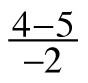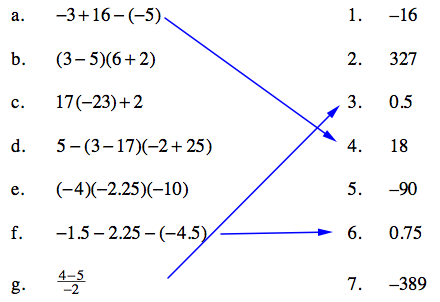Home > CCA > Chapter A > Lesson A.1.7 > ProblemA-75

A-75.

CALCULATOR CHECK

Use your scientific calculator to compute the value of each expression in the left-hand column below. Match each result to an answer in the right-hand column. A-75 HW eTool (Desmos). Desmos Accessibility Homework Help ✎

1. $−3+16−(−5)$

1. $-16$

1. $(3−5)(6+2)$

1. $327$

1. $17(−23)+2$

1. $0.5$

1. $5−(3−17)(−2+25)$

1. $18$

1. $(−4)(−2.25)(−10)$

1. $-90$

1. $−1.5−2.25−(−4.5)$

1. $0.75$

1.1. $-389$

Remember to be cautious when entering negative signs.
A few matches have been made below, so that you can check some of your work.Use the eTool below to compute the value of each expression.
Click the link at right for the full version of the eTool: CCA A-75 HW eTool